### COSMOLOGY, INFLATIONARY UNIVERSE

Robert H. Brandenberger

The inflationary universe is a cosmological model in which there is a period during the very early universe when the volume of space expands exponentially. A period of inflation may arise when matter is described by particle physics (instead of by an ideal gas) in the early universe. Inflationary models provide potential solutions to some of the problems of standard cosmological models. They also provide a mechanism which produces energy density fluctuations which can seed galaxies and other structures in the universe.

### MODELS

The inflationary universe may arise when one couples particle physics and classical general relativity, in particular when one describes matter in terms of fields. It is known that field theory describes the interaction of particles very well at high energies and temperatures when the ideal gas description of matter breaks down. Hence, the cosmological models obtained in this way will give a better description of the early universe than the classical models.

When describing matter in terms of fields, it is possible to obtain equations of state with negative pressure. For a scalar field (a field which does not change under Lorentz transformations)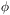(x, t), three terms contribute to the energy densityand pressure p - the kinetic energy density, the spatial gradient energy, and the contribution of a potential energy term V():= 1/2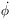2 + 1/2 R-2 (t) (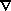)2 + V(),

p = 1/22 - 1/6 R-2 (t) ()2 - V().

Here, R (t) is the scale factor of the universe. If(x, t) is homogeneous and static and V() is positive, the equation of state is p = -. Hence, using the Einstein equations for a Friedmann universe, one obtains exponential increase of the scale factor (the de Sitter phase).

In some models,is homogeneous but time dependent. In this case, the equation of state is no longer p = -. However, it is still possible for such a cosmological model to solve the homogeneity and flatness problem (see the following), provided p < -1/3. This is the condition for R (t) to increase more rapidly than the Hubble radius H-1 (t), where

H (t) =(t) / R (t)

Models with -< p < -1/3are said to give rise to to give rise to generalized inflation.

Scaler fields arise in many particle physics models. They are often used to break gauge symmetries. In this case, a potential of the form of curve (a) in Fig. 1 is required. New inflationary universe models take such a potential and assume that at very early times(x)0.(x) will slowly roll towards one of the of the minimum energy values ±. If the potential is very flat, then it will take the field a long time to reach ±. During most of the rolling phase, p-and the universe expands exponentially.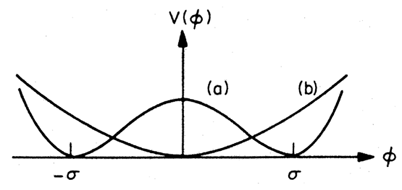Figure 1. A sketch of the potential V() postulated in new inflationary universe models (a) and for chaotic inflation (b).

Constraints stemming from the magnitude of density perturbations produced during inflation requires the coupling constant which determines the flatness of V() to be so small that the initial condition(x)0 can no longer be justified. This is taken into account in the chaotic inflationary universe. In this case, either potential (curve a or b of Fig. 1) can be chosen. The part of the universe inside our horizon is assumed to stem from a region in space where initially(x) was homogeneous over a few Hubble volumes and in addition>> mpl (where mpl stands for the Planck mass). In this case,(x) will slowly roll towards=(or= 0 in the case of curve b in Fig. 1), its motion being damped by the expansion of the universe. Again, p-and inflation occurs.

### HOMOGENEITY AND FLATNESS PROBLEMS

Inflationary models provide a potential explanation of the flatness of the universe and of its homogeneity on large scales. Homogeneity follows from the observed isotropy of the microwave background radiation to less than 1 part in 104. The flatness can be quantified by comparing the present energy densitywith the energy densityc for a flat (K = 0) universe. The ratio=/c is most likely between 0.1 and 1.

In classical cosmology, the isotropy of the microwave background has no causal explanation, because the radiation detected in different directions on the sky comes from patches of the last scattering surface, which at last scattering trec could not have been in causal contact. Similarly, in classical cosmology= 1 is an unstable fixed point of an expanding universe. Thus, havingclose to 1 today implies thathad to be tuned to almost= 1 with an extreme accuracy at early times.

As illustrated in Figure 2, in inflationary models the horizon expands exponentially during the period of inflation. Provided inflation lasts for more than a few Hubble expansion times H-1 (t), the horizon at teq is larger than the surface at trec from which the microwave radiation reaches us.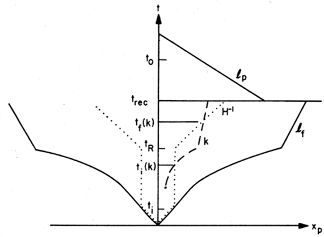Figure 2. A sketch of the cosmology on inflationary universe models. The phase of exponential expansion lasts from ti to tR. Before and after, the universe is dominated by radiation. The horizon lf (t) expands exponentially in the de Sitter phase. This provides a solution of the homogeneity problem: at recombination trec, the horizon is much larger than the domain lp for which we observe isotropy of the microwave background. Also shown in this sketch of physical distance xp versus time t is how comoving scales k exit the Hubble radius at time ti (k) during the de Sitter phase and reenter at tf (k) during the period of radiation dominance. t0 is the present time.

Similarly, inflation solves the flatness problem. During the period of inflation, |- 1| decreases exponentially. Thus,in the range between 0.1 and 1 today no longer requires unnatural fine tuning in the very early universe.

### INFLATION AND DENSITY PERTURBATIONS

Inflationary models provide a possible mechanism for generating the primordial density perturbations which can lead to structure formation. The simplest inflationary models give a scale invariant spectrum of linear, adiabatic random phase perturbations. Scale invariant means that the root-mean-square mass perturbation on a fixed comoving scale has a value which is independent of the scale when measured at the time when the scale equals the Hubble radius.

The basic mechanism is illustrated in Figure 2. All comoving scales originate inside the Hubble radius (causal horizon) during the de Sitter phase. Hence, it is possible to obtain a causal explanation of the origin of perturbations.

Quantum fluctuations in the de Sitter phase which arise on all comoving scales are frozen in as classical perturbations on scale k when the wavelength k-1 equals the Hubble radius at time ti (k). Between ti (k) and tf (k), the time when the wavelength ``reenters'' the Hubble radius, the mass perturbations grow according to linear gravitational perturbation theory and increase by a constant factor. Since the amplitude of the perturbations is k independent at ti (k), a scale-invariant spectrum is generated.

The amplitude of the spectrum of density perturbations depends on the coupling constants in the particle physics model. In order not to give a too-large amplitude, sell-coupling constants in V() typically must be tuned to be smaller than 10-12. This is a serious problem which affects most inflationary models.

### RECENT RESULTS

The inflationary universe has become a paradigm in search of a concrete realization. There have been attempts to obtain inflation in supergravity and superstring models, in theories with modified gravity or with extra dimensions. Extended inflation is a new attempt to use a dynamical Brans-Dicke scalar field to obtain generalized inflation.

Chaotic inflation is at present the most developed model. It predicts that on scales much larger than our present horizon, the universe will consist of many very different, almost disconnected miniuniverses.

In models in which more than one scalar field is important during the period of exponential expansion, it is possible to obtain deviations from scale invariance, isothermal perturbations, and even non-Gaussian fluctuations.